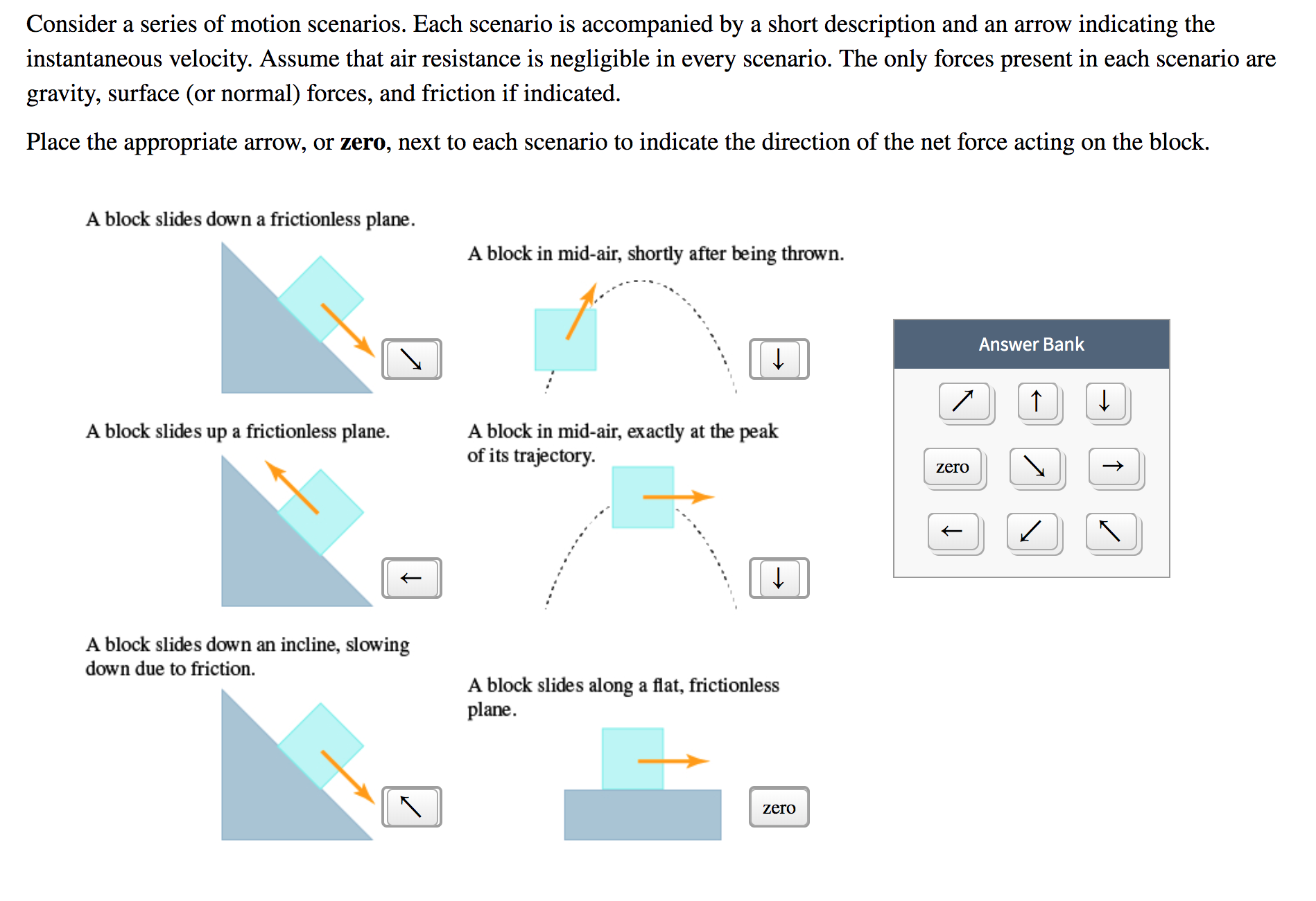# indicating theinstantaneous velocity. Assume that air resistance is negligible in every scenario. The only forces present in each scenario areConsider a series of motion scenarios. Each scenario is accompanied by a short description and an arrowассgravity, surface (or normal) forces, and friction if indicated.Place the appropriate arrow, or zero, next to each scenario to indicate the direction of the net force acting on the block.A block slides down a frictionless plane.A block in mid-air, shortly after being thrownAnswer BankLA block in mid-air, exactly at the peakof its trajectoryA block slides up a frictionless plane.zeroA block slides down an incline, slowingdown due to friction.A block slides along a flat, frictionlessplanezero

Question

Consider a series of motion scenarios. Each scenario is accompanied by a short description and an arrow indicating the instantaneous velocity. Assume that air resistance is negligible in every scenario. The only forces present in each scenario are gravity, surface (or normal) forces, and friction if indicated.help_outlineImage Transcriptioncloseindicating the instantaneous velocity. Assume that air resistance is negligible in every scenario. The only forces present in each scenario are Consider a series of motion scenarios. Each scenario is accompanied by a short description and an arrow асс gravity, surface (or normal) forces, and friction if indicated. Place the appropriate arrow, or zero, next to each scenario to indicate the direction of the net force acting on the block. A block slides down a frictionless plane. A block in mid-air, shortly after being thrown Answer Bank L A block in mid-air, exactly at the peak of its trajectory A block slides up a frictionless plane. zero A block slides down an incline, slowing down due to friction. A block slides along a flat, frictionless plane zero fullscreen
check_circleExpert Solution
Step 1

Normal force always acts perpendicular to the surface.

Step 2
• For the block sliding down a frictionless plane, normal force and the component of gravitational force perpendicular to the plane cancels each other. The block moves at a constant speed. Thus, the only force is the force due to the component of gravitational force along the plane.
• For a block in the mid-air, shortly after it is thrown, it is the only under the influence of gravitational force which points directly downwards.
• For the block sliding down a frictionless plane, normal force and the component of gravitational force perpendicular to the plane cancels each other. The block moves at a constant speed. Thus, the only force is the force due to the component of gravitational force along the plane.
• For a block in the mid-air, at the...

### Want to see the full answer?

See Solution

#### Want to see this answer and more?

Solutions are written by subject experts who are available 24/7. Questions are typically answered within 1 hour*

See Solution
*Response times may vary by subject and question
Tagged in

### Physics